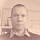# Data Science: Computation of Eigenvectors — Power Method.

This story is part of my Data Science series.

To exactly compute the eigenvalue/eigenvectors pairs, there exists several methods. One of this is the so called power method at which theory and implementation this article is devoted.

## Implementation:

The implementation will proceed what we have started in the previous story (see here).## An Introduction into RustThe power method is implemented easily like so:

`pub fn compute_ev_power_method(a: &Array2<f64>) -> (Array1<f64>, f64) {    let mut x = Array1::ones(a.nrows());    let max_iter = 10;    for _ in 0..max_iter {        x = a.dot(&x);        x /= *x.mapv(f64::abs).max().unwrap();    }    let lambda = &x.t().dot(&a.dot(&x)) / &x.t().dot(&x);     (x, lambda)}`

Of course, in practice one would apply in addition some convergence criterion to break out early from the loop. For instance `|Ax — lambda*x| < epsilon`.

Our complete program now looks like this:

`let data = load_data();let cov = compute_covariance(&data);let ev_circles = compute_ev_circles(&cov);let (e_vec, e_val) = compute_ev_power_method(&cov);println!("{}", e_val);`

This finds the eigenvalue `13.242`.

Having this, the question arises how to obtain the remaining eigenvalue/eigenvector pairs. More on this, in our next story.